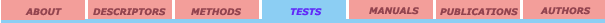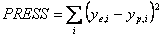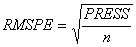External validation set Leave-One-Out crossvalidation Leave-Many-Out crossvalidation Randomization test

## External validation set

The easiest method of the correlation testing is use of an external validation set.  In this method, the correlation is used for predict a property value for a chemical structure that was not used in the creation of the correlation; some test statistics are calculated for the external dataset; the difference between the test statistics in the training and validation datasets is a measure of the reliability of the correlation. The widely used measure is the prediction error sum of squares (PRESS) and is defined aswhere ye,i are experimental values of the property and   yp,i  are predicted values for external validation test.

Often the RMSPE criterion is prefered:because it gives error  on a ‘per compound’ basis. 

The method is a particular case of the leave-many-out cross-validation method.

References:

1. Stone M.; Jonathan P. Statistical Thinking and Technique for QSAR and related studies. 1. Genaral Theory J. Chemometrics 1993, 7, 455-475.University of Florida 2001. All rights reserved